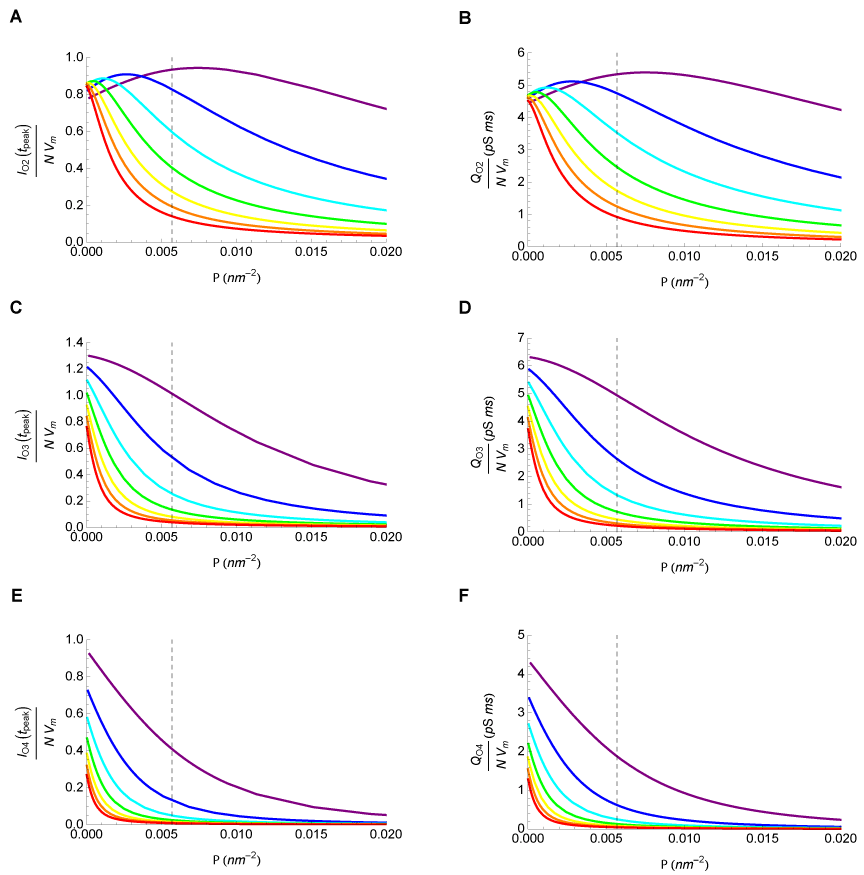Figure 8: Receptor-limited diffusion case: Contribution of the receptor’s open states on peak current and total charge. The individual contributions of the three open states, O2, O3 and O4, to the peak current and total charge were calculated as a function of receptor density. The graphs illustrate the relationships for the following radial dimensions: rPSD=42 nm (purple), rPSD=62 nm (blue), r=82PSD nm (cyan), rPSD=102 nm (green), rPSD=122 nm (yellow), rPSD=142 nm (orange) and rPSD=162 nm (red). The dashed line corresponds to the maximal receptor density for the AMPA receptor. (A) O2 Current. (B) O2 Charge. (C) O3 Current. (D) O3 Charge. (E) O4 Current. (F) O4 Charge.